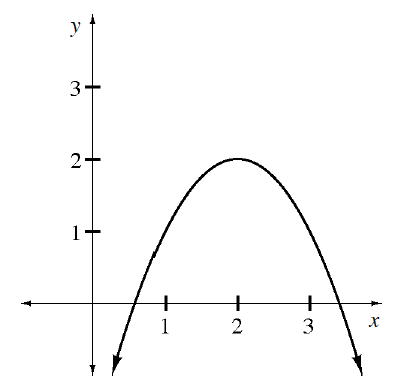### Home > APCALC > Chapter 11 > Lesson 11.3.1 > Problem11-85

11-85.

Multiple Choice: The graph of $y = f(x)$ is shown at right. The function is twice differentiable. Examine the graph and decide which statement below is true. Homework Help ✎

1. $f(2) < f ′(2) < f ″(2)$

1. $f(2) < f ″(2) < f ′(2)$

1. $f ′(2) < f(2) < f ″(2)$

1. $f ″(2) < f(2) < f ′(2)$

1. $f ″(2) < f ′(2) < f(2)$$f(2) = ?$
$f(2)$ is a maximum, so $f′(2) = ?$
The graph is concave down, so $f″(2)$ is ____.